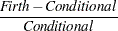## Example 53.12 Firth’s Penalized Likelihood Compared with Other Approaches

Firth’s penalized likelihood approach is a method of addressing issues of separability, small sample sizes, and bias of the parameter estimates. This example performs some comparisons between results from using the FIRTH option to results from the usual unconditional, conditional, and exact logistic regression analyses. When the sample size is large enough, the unconditional estimates and the Firth penalized-likelihood estimates should be nearly the same. These examples show that Firth’s penalized likelihood approach compares favorably with unconditional, conditional, and exact logistic regression; however, this is not an exhaustive analysis of Firth’s method. For more detailed analyses with separable data sets, see Heinze (2006, 1999) and Heinze and Schemper (2002).

### Comparison on 2x2 Tables with One Zero Cell

A 22 table with one cell having zero frequency, where the rows of the table are the levels of a covariate while the columns are the levels of the response variable, is an example of a quasi-completely separated data set. The parameter estimate for the covariate under unconditional logistic regression will move off to infinity, although PROC LOGISTIC will stop the iterations at an earlier point in the process. An exact logistic regression is sometimes performed to determine the importance of the covariate in describing the variation in the data, but the median-unbiased parameter estimate, while finite, might not be near the true value, and one confidence limit (for this example, the upper) is always infinite.

The following DATA step produces 1000 different 22 tables, all following an underlying probability structure, with one cell having a near zero probability of being observed:

```%let beta0=-15;
%let beta1=16;
data one;
keep sample X y pry;
do sample=1 to 1000;
do i=1 to 100;
X=rantbl(987987,.4,.6)-1;
xb= &beta0 + X*&beta1;
exb=exp(xb);
pry= exb/(1+exb);
cut= ranuni(393993);
if (pry < cut) then y=1; else y=0;
output;
end;
end;
run;
```

The following statements perform the bias-corrected and exact logistic regression on each of the 1000 different data sets, output the odds ratio tables by using the ODS OUTPUT statement, and compute various statistics across the data sets by using the MEANS procedure:

```ods exclude all;
proc logistic data=one;
by sample;
class X(param=ref);
model y(event='1')=X / firth clodds=pl;
ods output cloddspl=firth;
run;
proc logistic data=one exactonly;
by sample;
class X(param=ref);
model y(event='1')=X;
exact X / estimate=odds;
ods output exactoddsratio=exact;
run;
ods select all;
proc means data=firth;
var LowerCL OddsRatioEst UpperCL;
run;
proc means data=exact;
var LowerCL Estimate UpperCL;
run;
```

The results of the PROC MEANS statements are summarized in Table 53.14. You can see that the odds ratios are all quite large; the confidence limits on every table suggest that the covariate X is a significant factor in explaining the variability in the data.

Table 53.14 Odds Ratio Results

Method

Mean Estimate

Standard Error

Minimum Lower CL

Maximum Upper CL

Firth

231.59

83.57

10.40

111317

Exact

152.02

52.30

8.82### Comparison on Case-Control Data

Case-control models contain an intercept term for every case-control pair in the data set. This means that there are a large number of parameters compared to the number of observations. Breslow and Day (1980) note that the estimates from unconditional logistic regression are biased with the corresponding odds ratios off by a power of 2 from the true value; conditional logistic regression was developed to remedy this.

The following DATA step produces 1000 case-control data sets, with pair indicating the strata:

```%let beta0=1;
%let beta1=2;
data one;
do sample=1 to 1000;
do pair=1 to 20;
ran=ranuni(939393);
a=3*ranuni(9384984)-1;
pdf0= pdf('NORMAL',a,.4,1);
pdf1= pdf('NORMAL',a,1,1);
pry0= pdf0/(pdf0+pdf1);
pry1= 1-pry0;
xb= log(pry0/pry1);
x= (xb-&beta0*pair/100) / &beta1;
y=0;
output;
x= (-xb-&beta0*pair/100) / &beta1;
y=1;
output;
end;
end;
run;
```

Unconditional, conditional, exact, and Firth-adjusted analyses are performed on the data sets, and the mean, minimum, and maximum odds ratios and the mean upper and lower limits for the odds ratios are displayed in Table 53.15. Warning: Due to the exact analyses, this program takes a long time and a lot of resources to run. You might want to reduce the number of samples generated.

```ods exclude all;
proc logistic data=one;
by sample;
class pair / param=ref;
model y=x pair / clodds=pl;
ods output cloddspl=oru;
run;
data oru;
set oru;
if Effect='x';
rename lowercl=lclu uppercl=uclu oddsratioest=orestu;
run;
proc logistic data=one;
by sample;
strata pair;
model y=x / clodds=wald;
ods output cloddswald=orc;
run;
data orc;
set orc;
if Effect='x';
rename lowercl=lclc uppercl=uclc oddsratioest=orestc;
run;
proc logistic data=one exactonly;
by sample;
strata pair;
model y=x;
exact x / estimate=both;
ods output ExactOddsRatio=ore;
run;
proc logistic data=one;
by sample;
class pair / param=ref;
model y=x pair / firth clodds=pl;
ods output cloddspl=orf;
run;
data orf;
set orf;
if Effect='x';
rename lowercl=lclf uppercl=uclf oddsratioest=orestf;
run;
data all;
merge oru orc ore orf;
run;
ods select all;
proc means data=all;
run;
```

You can see from Table 53.15 that the conditional, exact, and Firth-adjusted results are all comparable, while the unconditional results are several orders of magnitude different.

Table 53.15 Odds Ratio Estimates

Method

N

Minimum

Mean

Maximum

Unconditional

1000

0.00045

112.09

38038

Conditional

1000

0.021

4.20

195

Exact

1000

0.021

4.20

195

Firth

1000

0.018

4.89

71

Further examination of the data set all shows that the differences between the square root of the unconditional odds ratio estimates and the conditional estimates have mean –0.00019 and standard deviation 0.0008, verifying that the unconditional odds ratio is about the square of the conditional odds ratio. The conditional and exact conditional odds ratios are also nearly equal, with their differences having mean 3E–7 and standard deviation 6E–6. The differences between the Firth and the conditional odds ratios can be large (mean 0.69, standard deviation 5.40), but their relative differences,, have mean 0.20 with standard deviation 0.19, so the largest differences occur with the larger estimates.